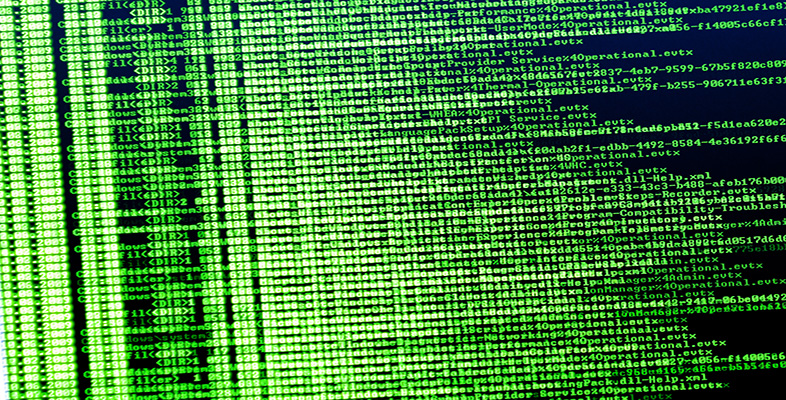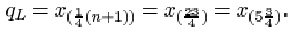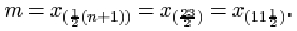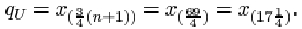Exploring data: Graphs and numerical summaries

Start this free course now. Just create an account and sign in. Enrol and complete the course for a free statement of participation or digital badge if available.

Free course

# 5.6.3 Interquartile range for the SIRDS data

For the 23 infants who survived SIRDS, the lower quartile is qL=1.720 kg, and the upper quartile is qU=2.830 kg. Thus the interquartile range (in kg) is

qUqL = 2.830 – 1.720 = 1.110.

## Activity 10: More on the SIRDS data

Find the lower and upper quartiles, and the interquartile range, for the birth weight data on those children with SIRDS who died. The ordered data are in Table 9.

### Solution

The lower quartile birth weight (in kg) for the 27 children who died is given by

qL = x (¼(n+1)) = x (7) = 1.230.

The upper quartile birth weight (in kg) is

qU = x (¾(n+1)) = x (21) = 2.220.

The interquartile range (in kg) is

qUqL = 2.200 – 1.230 = 0.970.

## Activity 11: Chondrite meteors

Find the median, the lower and upper quartiles, and the interquartile range for the data in Table 11, which give the percentage of silica found in each of 22 chondrite meteors. (The data are ordered.)

### Table 11 Silica content of chondrite meteors

 20.77 22.56 22.71 22.99 26.39 27.08 27.3 27.33 27.57 27.81 28.69 29.36 30.25 31.89 32.88 33.23 33.28 33.4 33.52 33.83 33.95 34.82

(Good, I.J. and Gaskins, R.A. (1980) Density estimation and bump-hunting by the penalized likelihood method exemplified by scattering and meteorite data. J. American Statistical Association, 75, 42–56.)

### Solution

For the silica data, the sample size n is 22. The lower quartile isSo qL is three-quarters of the way between

x (5)=26.39 and x (6)=27.08. That is

qL = 26.39 + ¾(27.08 – 26.39) = 26.9075,

or approximately 26.91. The sample median isThis is midway between x (11)=28.69 and x (12)=29.36. That is 29.025, or approximately 29.03.

The upper quartile isSo qU is one-quarter of the way between x (17)=33.28 and x (18)=33.40. That is

qU = 33.28 + ¼(33.40 – 33.28) = 33.31

The interquartile range is

qUqL = 33.31 – 26.9075 = 6.4025,

or approximately 6.40.

M248_1